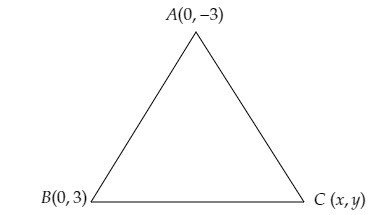# If $(0, -3)$ and $(0, 3)$ are the two vertices of an equilateral triangle, find the coordinates of its third vertex.

Given:

$(0, -3)$ and $(0, 3)$ are the two vertices of an equilateral triangle.

To do:

We have to find the coordinates of its third vertex.

Solution:Let $A (0, -3)$ and $B (0, 3)$ be two vertices of the equilateral triangle and the coordinates of the third vertex be $C (x, y)$.

This implies,

$AB=BC=CA$

We know that,

The distance between two points $\mathrm{A}\left(x_{1}, y_{1}\right)$ and $\mathrm{B}\left(x_{2}, y_{2}\right)$ is $\sqrt{\left(x_{2}-x_{1}\right)^{2}+\left(y_{2}-y_{1}\right)^{2}}$.

Therefore,

$\mathrm{AC}=\mathrm{AB}$

$\Rightarrow (x-0)^{2}+(y+3)^{2}=(0-0)^{2}+(3+3)^{2}$

$\Rightarrow x^{2}+(y+3)^{2}=0+(6)^{2}=36$

$\Rightarrow x^{2}+y^{2}+6 y+9=36$

$\Rightarrow x^{2}+y^{2}+6 y=36-9=27$......(i)

$\mathrm{BC}=\mathrm{AB}$

$\Rightarrow (x-0)^{2}+(y-3)^{2}=36$

$\Rightarrow x^{2}+y^{2}+9-6 y=36$

$\Rightarrow x^{2}+y^{2}-6 y=36-9=27$........(ii)

From (i) and (ii),

$x^{2}+y^{2}+6 y=x^{2}+y^{2}-6 y$

$x^{2}+y^{2}+6 y-x^{2}-y^{2}+6 y=0$

$12 y=0$

$\Rightarrow y=0$

From (i),

$x^{2}+y^{2}+6 y=27$

$x^{2}+0+0=27$

$x=\pm \sqrt{9 \times 3}$

$=\pm 3 \sqrt{3}$

$x=\pm 3 \sqrt{3}$ and  $y=0$.

Therefore, the coordinates of the third point is $(3 \sqrt{3}, 0)$ or $(-3 \sqrt{3}, 0)$.

Updated on: 10-Oct-2022

727 Views